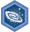## FANDOM

1,895 Pages

Pre-algebra: Negative numbers and coordinate planeDescription
Achieve mastery in all skills in Pre-algebra: Negative numbers and coordinate plane
Details
TypeEnergy point(s) awarded 0
Status Available
Disclosed?
Repeating?

This page contains exercises which pertain to the subcategory Pre-algebra: Negative numbers and coordinate plane. Mastering them awards user with the Challenge Patch Pre-algebra: Negative numbers and coordinate plane as well.

All items (12)

A
•Absolute value to find distance 2
•Adding negative numbers intro
C
•Comparing absolute values
F
•Finding absolute values
I
•Interpreting absolute value
•Interpreting negative numbers
M
•Multiplying and dividing negative numbers
N
•Negative numbers on the number line
•Negative numbers on the number line without reference to zero
•Number opposites
O
•Ordering small negative numbers
S
•Subtracting negative numbers intro